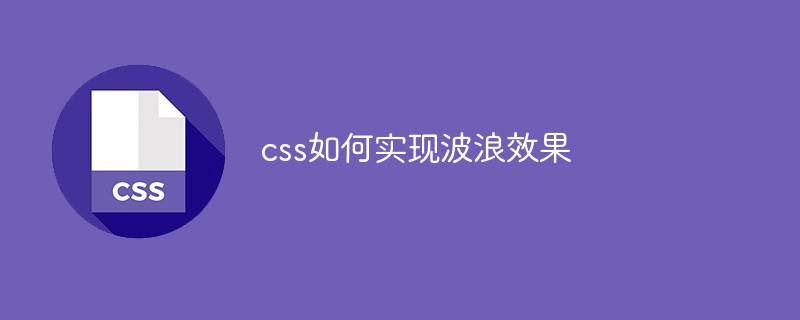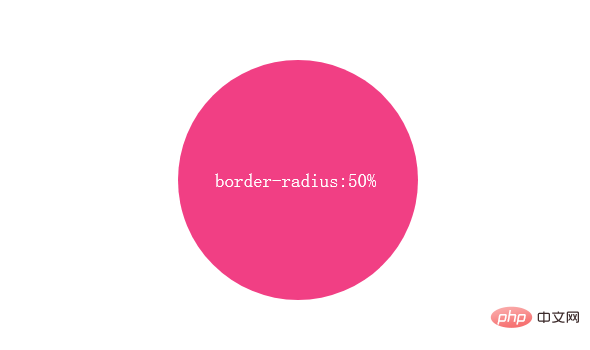# css如何实现波浪效果

css实现波浪效果的方法：首先创建一个HTML示例文件；然后创建一个正方形的div；接着通过添加“border-radius: 50%”属性实现圆形效果；最后通过animation属性使圆形滚动起来，用以达到波浪效果即可。## SVG 实现波浪效果```<svg width="200px" height="200px" version="1.1" xmlns="http://www.w3.org/2000/svg">
<text class="liquidFillGaugeText" text-anchor="middle" font-size="42px" transform="translate(100,120)" style="fill: #000">50.0%</text>
<!-- Wave -->
<g id="wave">
<path id="wave-2" fill="rgba(154, 205, 50, .8)" d="M 0 100 C 133.633 85.12 51.54 116.327 200 100 A 95 95 0 0 1 0 100 Z">
<animate dur="5s" repeatCount="indefinite" attributeName="d" attributeType="XML" values="M0 100 C90 28, 92 179, 200 100 A95 95 0 0 1 0 100 Z;
M0 100 C145 100, 41 100, 200 100 A95 95 0 0 1 0 100 Z;
M0 100 C90 28, 92 179, 200 100 A95 95 0 0 1 0 100 Z"></animate>
</path>
</g>
<circle cx="100" cy="100" r="80" stroke-width="10" stroke="white" fill="transparent"></circle>
<circle cx="100" cy="100" r="90" stroke-width="20" stroke="yellowgreen" fill="none" class="percentage-pie-svg"></circle>
</svg>```

## canvas 实现波浪效果```\$(function() {
let canvas = \$("canvas");
let ctx = canvas.getContext('2d');
let radians = (Math.PI / 180) * 180;
let startTime = Date.now();
let time = 2000;
let clockwise = 1;
let cp1x, cp1y, cp2x, cp2y;

// 初始状态
// ctx.bezierCurveTo(90, 28, 92, 179, 200, 100);
// 末尾状态
// ctx.bezierCurveTo(145, 100, 41, 100, 200, 100);

requestAnimationFrame(function waveDraw() {
let t = Math.min(1.0, (Date.now() - startTime) / time);

if(clockwise) {
cp1x = 90 + (55 * t);
cp1y = 28 + (72 * t);
cp2x = 92 - (51 * t);
cp2y = 179 - (79 * t);
} else {
cp1x = 145 - (55 * t);
cp1y = 100 - (72 * t);
cp2x = 41 + (51 * t);
cp2y = 100 + (79 * t);
}

ctx.clearRect(0, 0, 200, 200);
ctx.beginPath();
ctx.moveTo(0, 100);
// 绘制三次贝塞尔曲线
ctx.bezierCurveTo(cp1x, cp1y, cp2x, cp2y, 200, 100);
// 绘制圆弧
ctx.arc(100, 100, 100, 0, radians, 0);
ctx.fillStyle = "rgba(154, 205, 50, .8)";
ctx.fill();
ctx.save();

if( t == 1 ) {
startTime = Date.now();
clockwise = !clockwise;
}

requestAnimationFrame(waveDraw);
});
})
web前端开发学习Q-q-u-n： 767273102 ，分享开发工具，零基础，进阶视频教程，希望新手少走弯路```

## CSS实现波浪效果

#### 原理```width: 240px;
height: 240px;
background: #f13f84;
border-radius: 50%;``````width: 240px;
height: 240px;
background: #f13f84;
border-radius: 40%;``````@keyframes rotate{
from{transform: rotate(0deg)}
to{transform: rotate(359deg)}
}
.ripple{
width: 240px;
height: 240px;
background: #f13f84;
border-radius: 40%;
animation: rotate 3s linear infinite;
}```

#### 实现#### 相关文章推荐

• css怎么设置字体颜色• css样式有哪几种类型• div css怎么实现显示隐藏• css怎么设置微软雅黑• css怎么设置背景不动

### 全部评论我要评论

• 取消发布评论发送
• 1/1